Course Content

Classification with Python

##Train-test split. Cross validation

In the previous chapters, we built the models and predicted new values. But we have no idea how well the model performs and whether those predictions are trustworthy.

### Train-test split

To measure the model's performance, we need the subset of labeled data that the model had not seen. So we randomly split all the labeled data into training set and test set.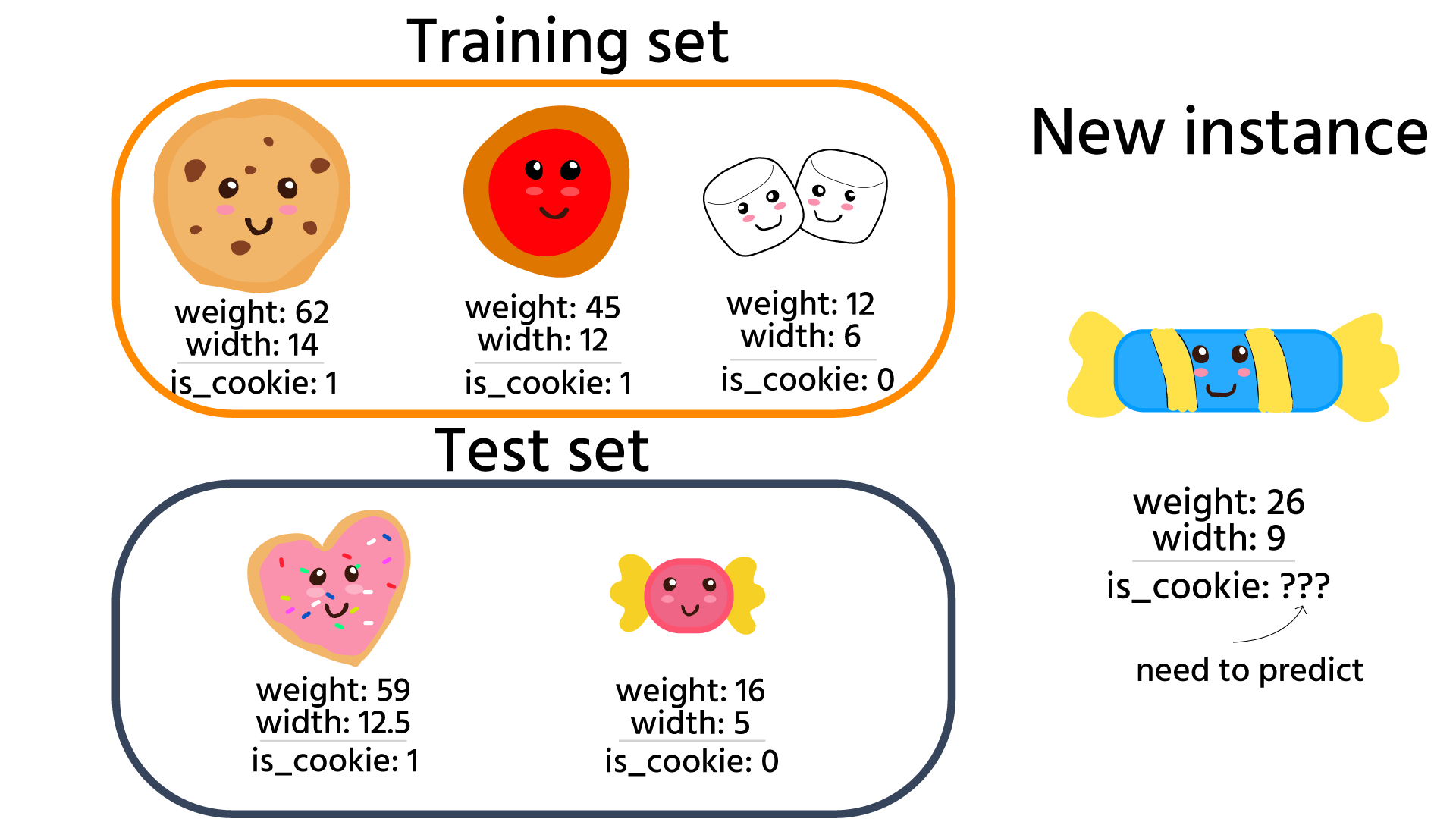This is achievable using the `train_test_split()` function of `sklearn`.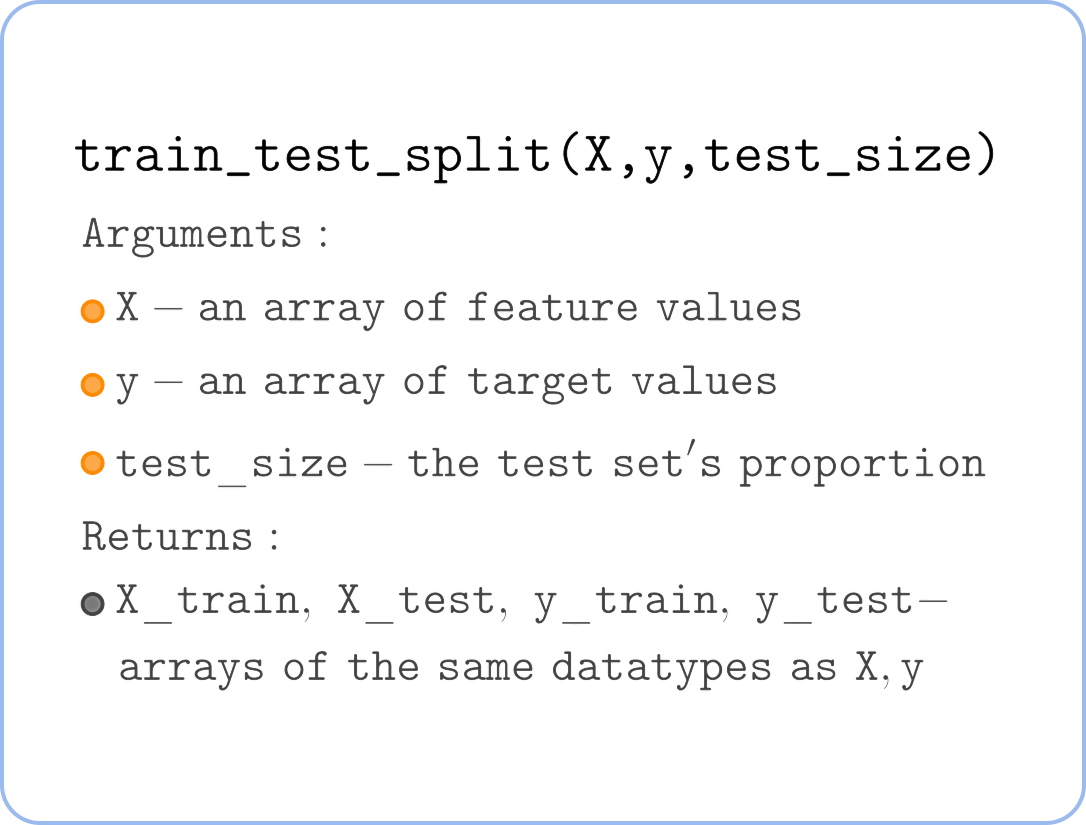Usually, you split the model around 70-90% for the training set and 10-30% for the test set. However, tens of thousands of test instances are more than enough, so there is no need to use even 10% if your dataset is large(millions of instances).
Now we can train the model using the training set and calculate its accuracy on the test set.But this approach has some flaws:

• We do not use all the available data for training, which could improve our model.
• Since we evaluate the model's accuracy on a small portion of data(test set), this accuracy score can be unreliable on smaller datasets (you can run the code above multiple times and see how the accuracy changes each time a new test set is sampled).

### Cross-validation

The cross-validation is designed for fighting those problems. Its idea is to shuffle the whole set, split it into 5 equal parts(folds), and run 5 iterations where you will use 4 parts for training and 1 as a test set.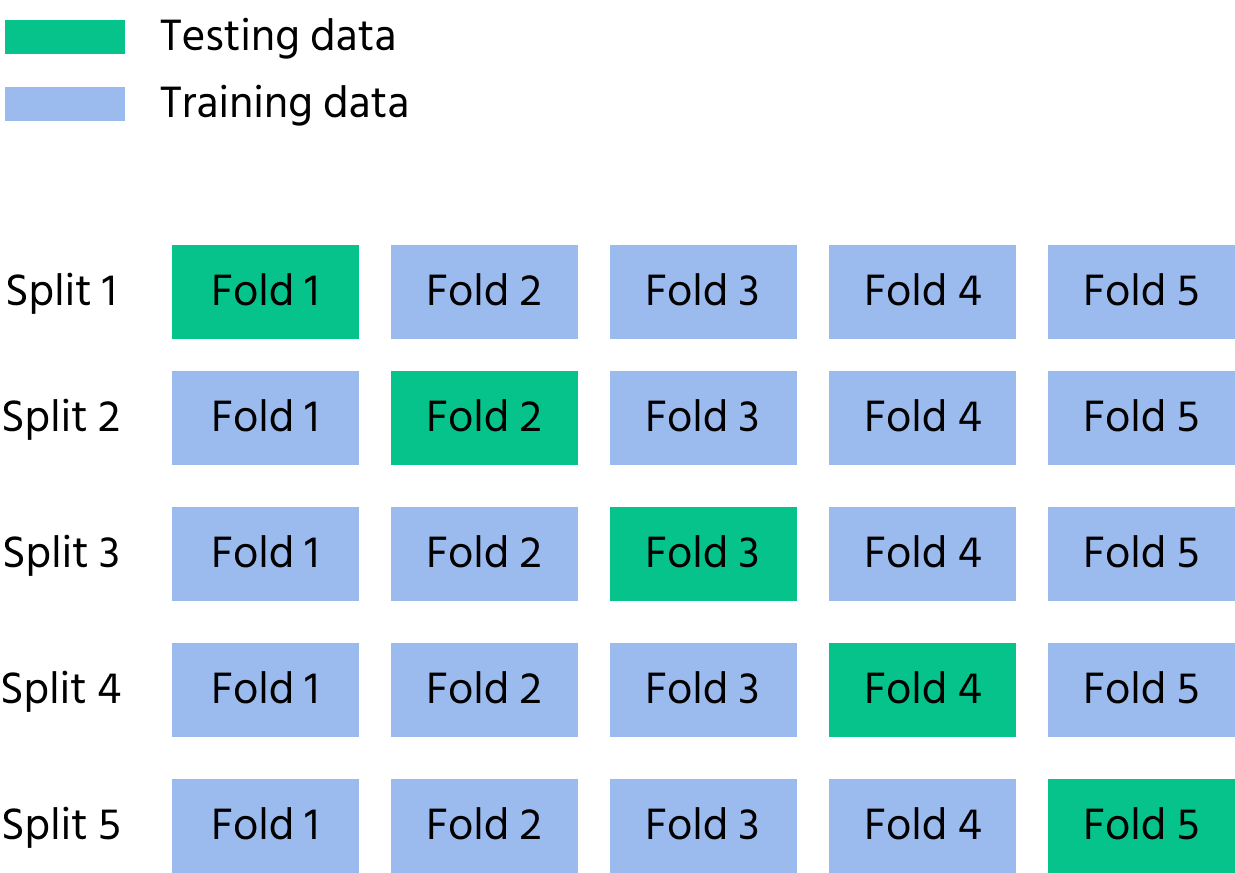So we train five models with little different datasets. At each, we calculate the test set accuracy. Once we've done that, we can take an average of those 5 accuracy scores, which will be our cross-validation accuracy score. It is more reliable since we calculated the accuracy score on all our data, just used five iterations for that.
Now we know how well the model performs and can re-train the model using the whole dataset.

Note

You can use the number of folds other than five. Say some number n. Then you will use one fold for the test set and n-1 for the training set. The following function makes it easy to configure such things.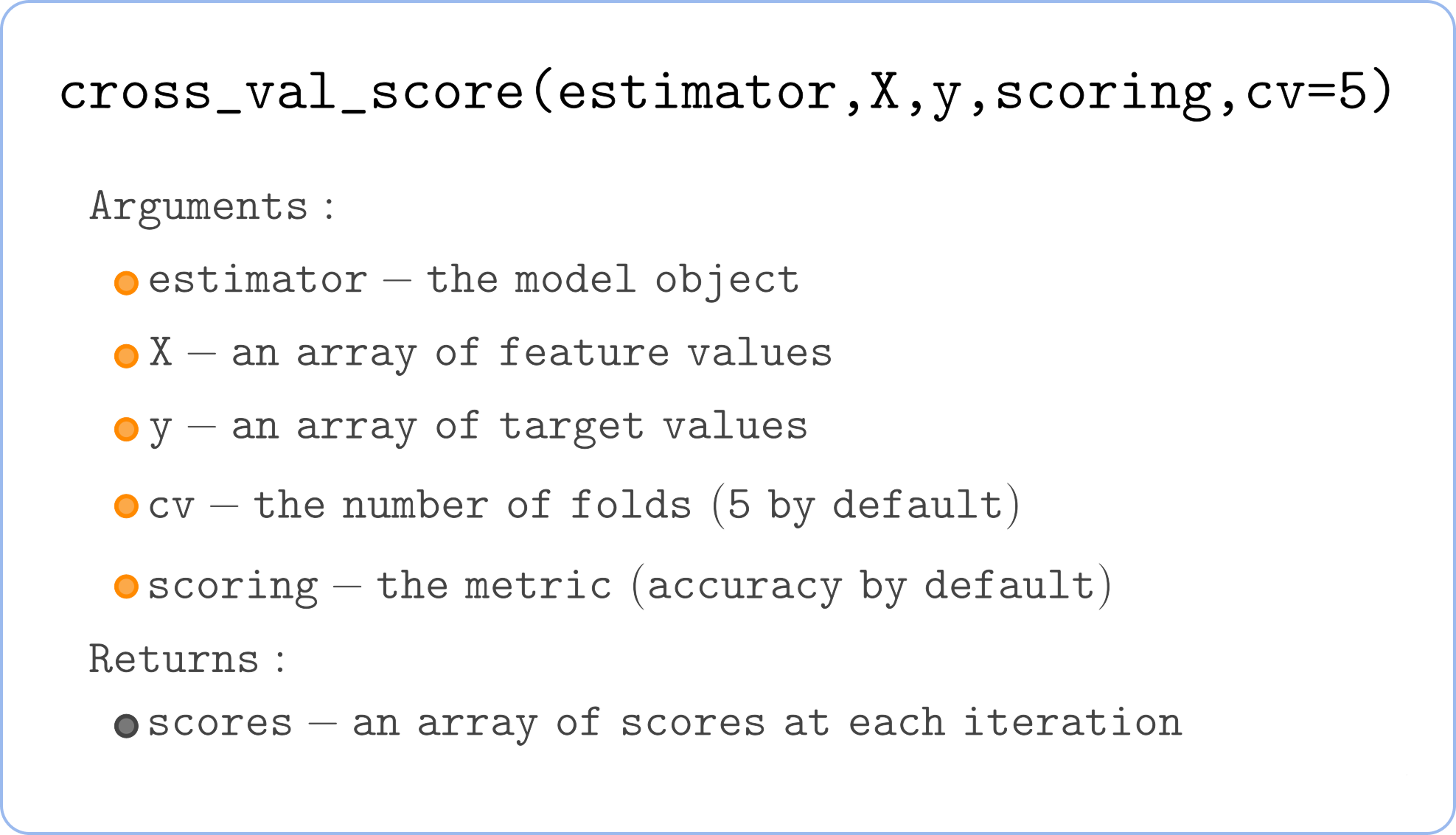Here is an example of usage:The score used by default for classification is accuracy: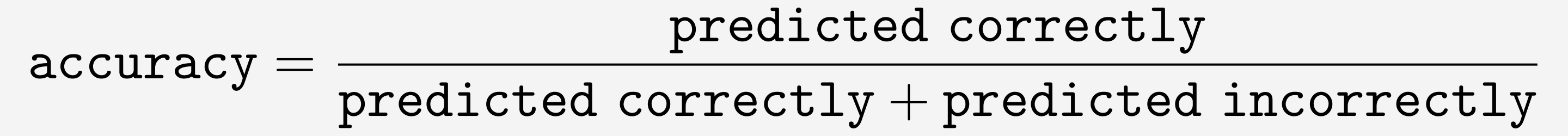So only around 75% of predictions are correct. But maybe with different `n_neighbors`, the accuracy will be better? It will! The following chapter covers choosing the `n_neighbors`(or k) with the highest cross-validation accuracy.

#Choose all the correct statements.

## Select a few correct answers

Everything was clear?

Section 1. Chapter 6